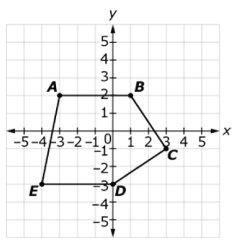# MAFS.912.G-GPE.2.7Archived StandardExport Print
Use coordinates to compute perimeters of polygons and areas of triangles and rectangles, e.g., using the distance formula.

### Remarks

Geometry - Fluency Recommendations

Fluency with the use of coordinates to establish geometric results, calculate length and angle, and use geometric representations as a modeling tool are some of the most valuable tools in mathematics and related fields.
General Information
Subject Area: Mathematics
Domain-Subdomain: Geometry: Expressing Geometric Properties with Equations
Cluster: Level 1: Recall
Cluster: Use coordinates to prove simple geometric theorems algebraically. (Geometry - Major Cluster) -

Clusters should not be sorted from Major to Supporting and then taught in that order. To do so would strip the coherence of the mathematical ideas and miss the opportunity to enhance the major work of the grade with the supporting clusters.

Date of Last Rating: 02/14
Status: State Board Approved - Archived
Assessed: Yes
Test Item Specifications

• Assessment Limits :
Items may require the use of the Pythagorean theorem.

Items may include convex, concave, regular, and/or irregular
polygons.

In items that require the student to find the area, the polygon must
be able to be divided into triangles and rectangles.

• Calculator :

Neutral

• Clarification :
Students will use coordinate geometry to find a perimeter of a
polygon.

Students will use coordinate geometry to find the area of triangles
and rectangles.

• Stimulus Attributes :
Items must be set in a real-world context.
• Response Attributes :
Items may require the student to apply the basic modeling cycle.

Items may require the student to use or choose the correct unit of
measure.

Items may require the student to find a dimension given the
perimeter or area of a polygon.

Sample Test Items (1)
• Test Item #: Sample Item 1
• Question:

Polygon ABCDE is shown on the coordinate grid.What is the perimeter, to the nearest hundredth of a unit, of polygon ABCDE?

• Difficulty: N/A
• Type: EE: Equation Editor

## Related Courses

This benchmark is part of these courses.
1200400: Foundational Skills in Mathematics 9-12 (Specifically in versions: 2014 - 2015, 2015 - 2022, 2022 and beyond (current))
1206300: Informal Geometry (Specifically in versions: 2014 - 2015, 2015 - 2022 (course terminated))
1206310: Geometry (Specifically in versions: 2014 - 2015, 2015 - 2022, 2022 and beyond (current))
1206320: Geometry Honors (Specifically in versions: 2014 - 2015, 2015 - 2022, 2022 and beyond (current))
1200700: Mathematics for College Algebra (Specifically in versions: 2014 - 2015, 2015 - 2022 (course terminated))
7912060: Access Informal Geometry (Specifically in versions: 2014 - 2015 (course terminated))
1206315: Geometry for Credit Recovery (Specifically in versions: 2014 - 2015, 2015 - 2022, 2022 and beyond (current))
7912065: Access Geometry (Specifically in versions: 2015 - 2022, 2022 and beyond (current))

## Related Access Points

Alternate version of this benchmark for students with significant cognitive disabilities.

## Related Resources

Vetted resources educators can use to teach the concepts and skills in this benchmark.

## Formative Assessments

Perimeter and Area of an Obtuse Triangle:

Students are asked to find the perimeter and area of an obtuse triangle given in the coordinate plane.

Type: Formative Assessment

Perimeter and Area of a Right Triangle:

Students are asked to find the perimeter and the area of a right triangle given in the coordinate plane.

Type: Formative Assessment

Perimeter and Area of a Rectangle:

Students are asked to find the perimeter and the area of a rectangle given in the coordinate plane.

Type: Formative Assessment

Pentagon’s Perimeter:

Students are asked to find the perimeter of a pentagon given in the coordinate plane.

Type: Formative Assessment

## Lesson Plans

My Geometry Classroom:

Students will learn how to find the area and perimeter of multiple polygons in the coordinate plane using the composition and decomposition methods, with the support of previous knowledge on the Distance Formula and Pythagorean Theorem. Students will complete a Geometry Classroom Floor Plan group activity. Students will do a short presentation to discuss their results that leads to the realization that polygons with the same perimeter can have different areas. Students will also complete an independent practice and submit an exit ticket at the end of the lesson.

Type: Lesson Plan

Just Plane Ol' Area!:

Students will construct various figures on coordinate planes and calculate the perimeter and area. Use of the Pythagorean theorem will be required.

Type: Lesson Plan

Going the Distance:

This lesson uses the Pythagorean Theorem to derive several iterations of the Distance Formula. The Distance Formula is then used to calculate the distance between two points on both directional maps and the Cartesian coordinate plane. Vocabulary relating to vectors is also introduced.

Type: Lesson Plan

## Perspectives Video: Professional/Enthusiast

This researcher knows where your cat lives! Watch how he uses coordinates and the distance formula to plot the location of hundreds of thousands of cats on a map.

Type: Perspectives Video: Professional/Enthusiast

## MFAS Formative Assessments

Pentagon’s Perimeter:

Students are asked to find the perimeter of a pentagon given in the coordinate plane.

Perimeter and Area of a Rectangle:

Students are asked to find the perimeter and the area of a rectangle given in the coordinate plane.

Perimeter and Area of a Right Triangle:

Students are asked to find the perimeter and the area of a right triangle given in the coordinate plane.

Perimeter and Area of an Obtuse Triangle:

Students are asked to find the perimeter and area of an obtuse triangle given in the coordinate plane.

## Student Resources

Vetted resources students can use to learn the concepts and skills in this benchmark.

## Parent Resources

Vetted resources caregivers can use to help students learn the concepts and skills in this benchmark.